" My girlfriend is from imagi nation. "

#  ML-Logistic regressionIt has been a long time after I wrote ML-Linear regression maybe because I am busy dealing with stuff concerning my graduation(enjoy parties). Anyway, I will catch up with my plan and update my blog as soon as I can.

# Definition

In classification problems, linear regression is not a good way to predict output Y. One of reasons is that hypothesis(θ) may be greater than 1 or less than 0 while the logistic regression could limit the output of h(θ) between 0 and 1.

We call it Logistic function/Sigmoid function: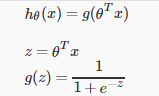The hypothesis output is similar to probability. Concretely, the h~θ~(x) =Probability(y=1|x;θ). So we want to predict if h~θ~(x) will greater or less than 0.5 to know output y is 0 or 1.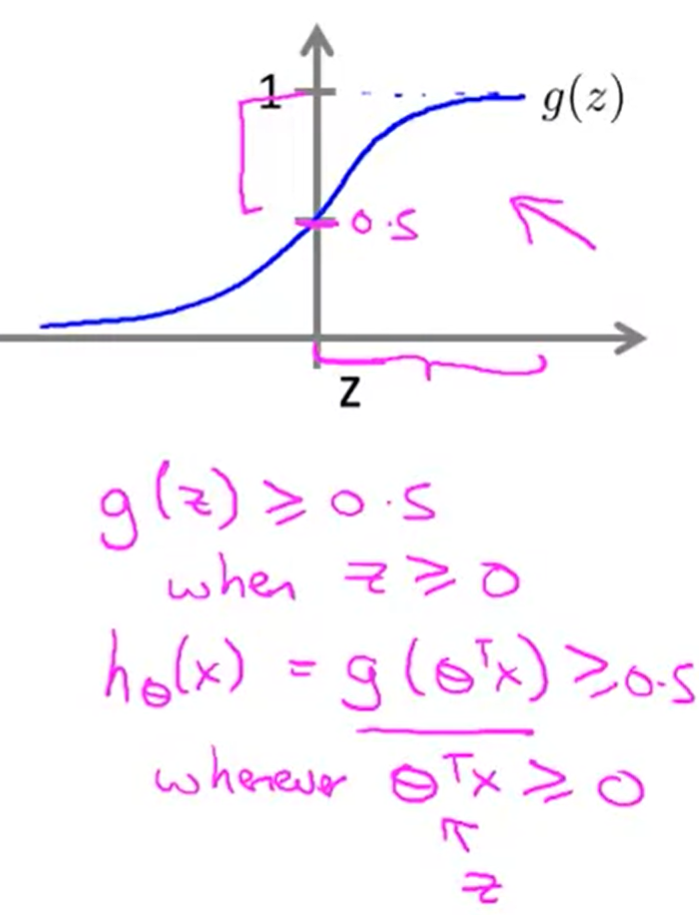As we can see the h~θ~(x)/g(z) in the picture, g(z) will equals 0.5 when Z equals 0. So the problem will become that predict if Z larger or smaller than 0.

The Decision Boundary can visualize this goal very well. Here are two examples: Linear decision boundary and non-linear decision boundary: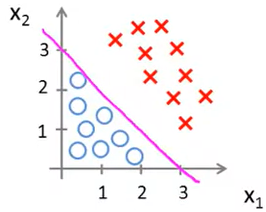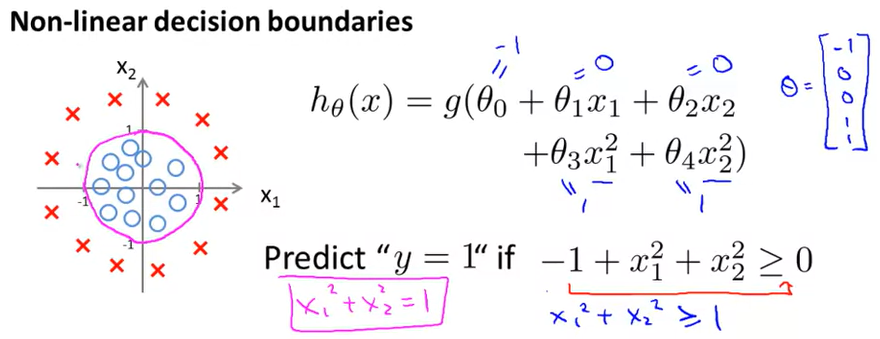Moreover, you can use different decision boundaries to predict output g(z) and it depends on what kind of function you use in Z.

# Cost Function

Next, as we have already know training set (x1,y1;x2,y2…x~n~,y~n~), we should choose a θi to decide our decision boundary which could fit the data set best. The logistic is as same as Linear regression—–build a cost function.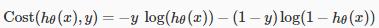or we could write this: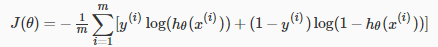To better understand this cost function, we should know why we use log function. If we plot -log[h(θ)] (when y=1) and -log[1-h(θ)] (when y=0), we could know the h(θ) range from 0 to 1 while the cost output range from 0 to infinite.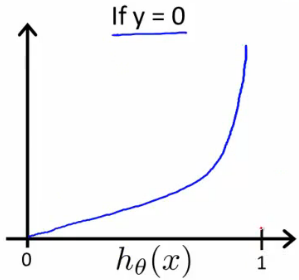We penalize the learning algorithm by a very, very large cost. And that’s captured by having this cost go to infinity if y equals 1 and h(θ) approaches 0.

Similar to linear regression, we can use gradient descent to calculate minimum of Cost function J(θ) and the main difference is just the definition of h(θ).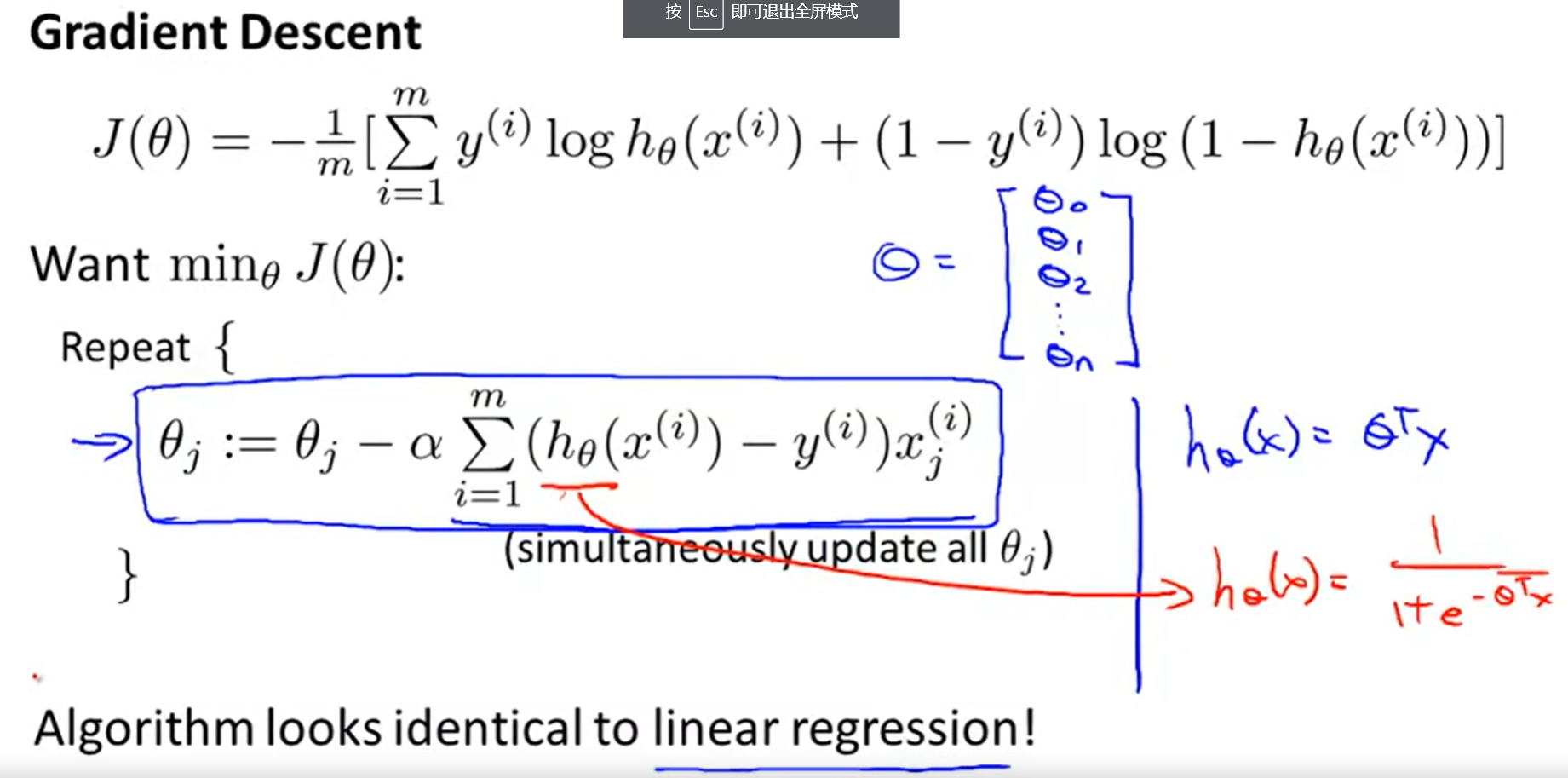Besides, many other algorithms could also calculate min J(θ). Some properties are mentioned below:

Algorithm Property
conjugate gradient /BFGS /L-BFGS Pros: No need to pick α; Faster than gradient descent Cons: complex

To better summarize Linear regression and Logistic regression, I made a table for comparing some key functions of them.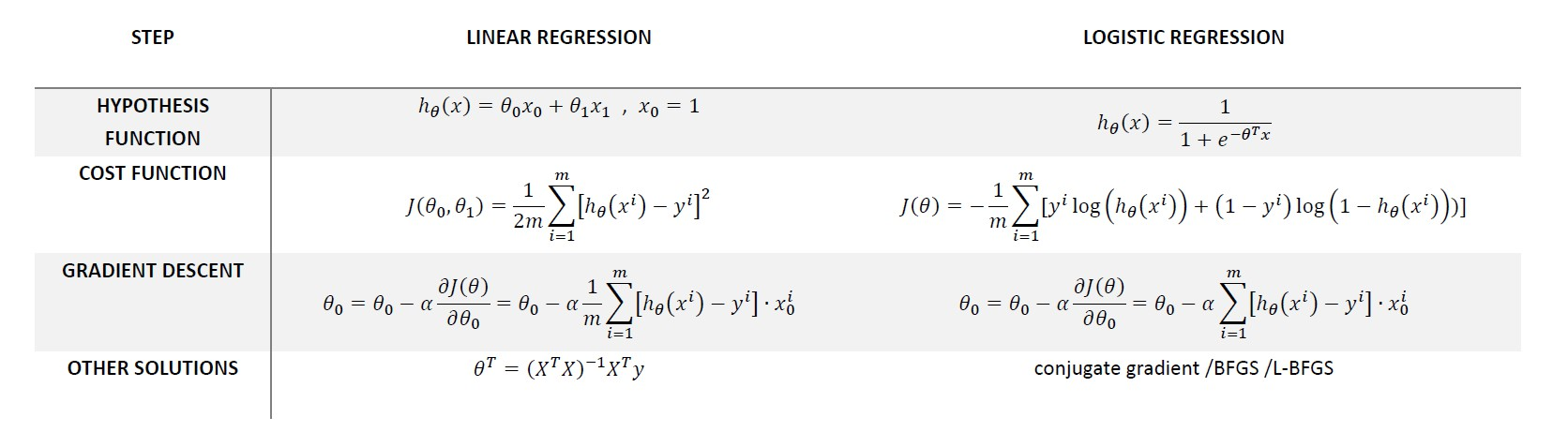I noticed some equation display errors on the website, I will fix it as soon as possible

• 725
• 1

### YOU MIGHT ALSO LIKE

0 0 vote
Article Rating
Subscribe1 评论
Inline Feedbacks